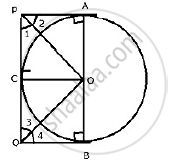Share

# Two Parallel Tangents of a Circle Meet a Third Tangent at Points P and Q. Prove that Pq Subtends a Right Angle at the Centre. - Mathematics

Course

#### Question

Two parallel tangents of a circle meet a third tangent at points P and Q. prove that PQ subtends a right angle at the centre.

#### SolutionJoin OP, OQ, OA, OB and OC.
In Δ OAP and Δ OCP

OA = OC (Radii of the same circle)
OP = OP (Common)
PA = PC (Tangents from P)
∴ By side – side – side criterion of congruence,
ΔOAP  ≅ Δ OCP (SSS postulate)
The corresponding parts of the congruent triangles are congruent.
⇒ ∠APO = ∠CPO     (cpct) ………….(i)
Similarly, we can prove that
∴ Δ OCQ  ≅  ΔOBQ
⇒ ∠ CQO = ∠BQO ……………(ii)
∴ ∠ APC = 2∠CPO and ∠CQB= 2∠CQO
But,
∠APC = ∠ CQB  = 180
(Sum of interior angles of a transversal)
∴ 2∠CPO + 2∠CQO = 180°

⇒ ∠CPO + ∠CQO = 90°
Now in ΔPOQ,
∠CPO  + ∠CQO + ∠POQ = 180°

⇒90° + ∠POQ  = 180°
∴ ∠ POQ  = 90°

Is there an error in this question or solution?